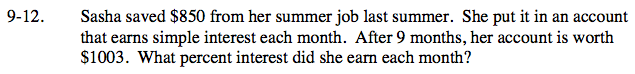Home > CC2 > Chapter 9 > Lesson 9.1.1 > Problem9-12

9-12.

Sasha saved $850 from her summer job last summer. She put it in an account that earns simple interest each month. After 9 months, her account is worth$1003. What percent interest did she earn each month? Homework Help ✎Find the amount of money Sasha gained over the 9 months (the interest).

$1003 −$850 = \$153

Use the equation I = Prt, where I is the interest, P is the principle (original amount she had), r is the rate (percent interest per month), and t is the time (number of months that have passed).

153 = (850) r (9) Solve for r.

$r=\frac{153}{850(9)}$

2% interest